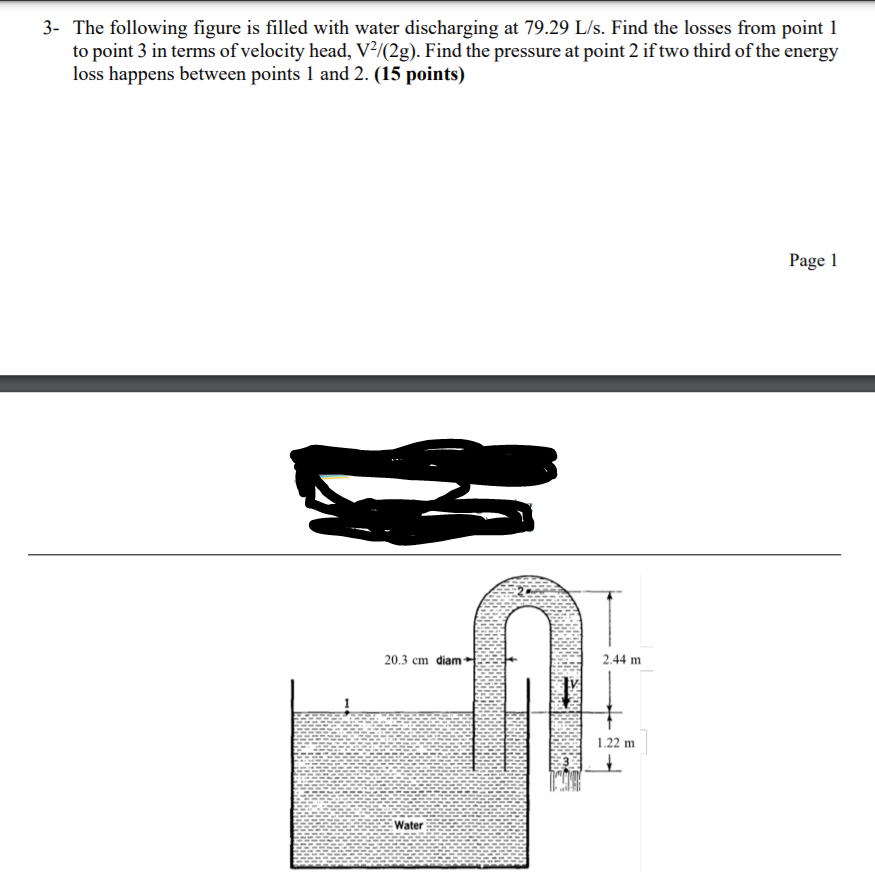# Question 3- The following figure is filled with water discharging at 79.29 L/s. Find the losses from point 1 to point 3 in terms of velocity head, V2/(2g). Find the pressure at point 2 if two third of the energy loss happens between points 1 and 2. (15 points) Page 1 20.3 cm diam 2.44 m 1.22 m WaterYAACMU The Asker · Civil EngineeringTranscribed Image Text: 3- The following figure is filled with water discharging at 79.29 L/s. Find the losses from point 1 to point 3 in terms of velocity head, V2/(2g). Find the pressure at point 2 if two third of the energy loss happens between points 1 and 2. (15 points) Page 1 20.3 cm diam 2.44 m 1.22 m Water
More
Transcribed Image Text: 3- The following figure is filled with water discharging at 79.29 L/s. Find the losses from point 1 to point 3 in terms of velocity head, V2/(2g). Find the pressure at point 2 if two third of the energy loss happens between points 1 and 2. (15 points) Page 1 20.3 cm diam 2.44 m 1.22 m Water